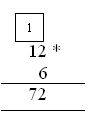Name: ___________________Date:___________________

 Email us to get an instant 20% discount on highly effective K-12 Math & English kwizNET Programs!

### Grade 3 - Mathematics1.12 Multiplication with One Digit - 3

 Method: Start with the single digit number. Multiply the single digit number and the number in ones place of the 2 digit number. If the result is more than 9, then write the tens digit of the result as a carry above the tens digit of the 2nd number. Multiply the single digit number and the number in tens place of the 2 digit number and add the carry to the product. Example: 6 x 12 = ?Single digit number is 6 and the two digit number is 12. 6 x 2 = 12. 12 is more than 9. Write 2 under ones place and carry 1 to the tens place. 6 x 1 = 6. Add carry to this result. 6 + 1 = 7. Write 7 below tens place. Answer: 72 Directions: Multiply the following and write at least 10 examples of your own for multiplication of double digit number by a single digit number.
 Q 1: 8 x 8 =Answer: Q 2: 3 x 12 =Answer: Question 3: This question is available to subscribers only! Question 4: This question is available to subscribers only!

#### Subscription to kwizNET Learning System costs less than \$1 per month & offers the following benefits:

• Unrestricted access to grade appropriate lessons, quizzes, & printable worksheets
• Instant scoring of online quizzes
• Progress tracking and award certificates to keep your student motivated
• Unlimited practice with auto-generated 'WIZ MATH' quizzes